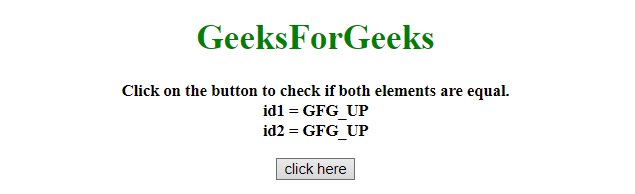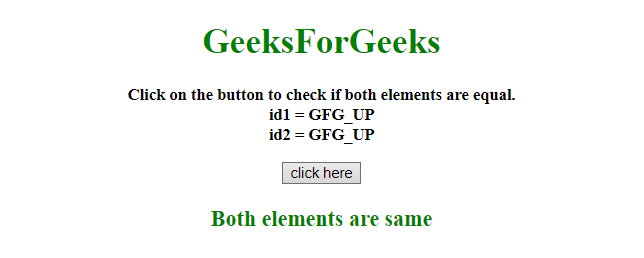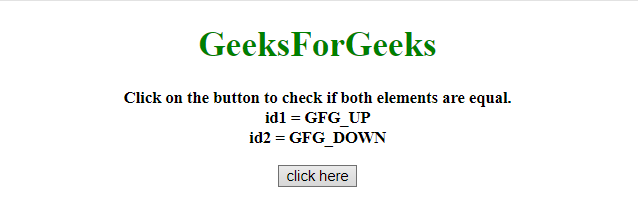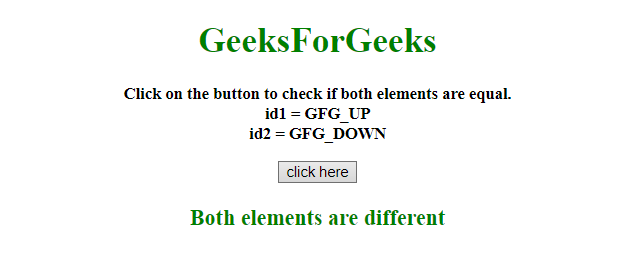# How to check two elements are same using jQuery/JavaScript ?

Given an HTML document containing two elements and the task is to check whether both elements are same or not with the help of JavaScript.

Approach 1: Use is() method to check both selected elements are same or not. It takes an element as argument and check if it is equal to the other element.

Example: This example implements the above approach.

 `   ` `<``html``>   ` ` `  `<``head``>  ` `    ``<``title``>  ` `       ``How to check two elements are same ` `       ``using jQuery/JavaScript? ` `    `` ` `     `  `    ``<``script` `src``= ` `"https://ajax.googleapis.com/ajax/libs/jquery/3.4.1/jquery.min.js"``> ` `    `` ` ` ` ` `  `<``body` `style` `= ``"text-align:center;"``>   ` `     `  `    ``<``h1` `style``=``"color:green;"``>   ` `        ``GeeksForGeeks   ` `    `` ` `     `  `    ``<``p` `id` `= ``"GFG_UP"` `style` `= ` `        ``"font-size: 15px; font-weight: bold;"``> ` `    `` ` `     `  `    ``<``button` `onclick` `= ``"GFG_Fun()"``> ` `        ``click here ` `    `` ` `     `  `    ``<``p` `id` `= ``"GFG_DOWN"` `style` `= ` `        ``"color:green; font-size: 20px; font-weight: bold;"``> ` `    `` ` `     `  `    ``<``script``>  ` `        ``var up = document.getElementById('GFG_UP'); ` `        ``var down = document.getElementById('GFG_DOWN'); ` `        ``var id1 = "GFG_UP"; ` `        ``var id2 = "GFG_UP"; ` `         `  `        ``up.innerHTML = "Click on the button to check if " ` `                ``+ "both elements are equal.<``br``>" + "id1 = " ` `                ``+ id1 + "<``br``>id2 = " + id2; ` `         `  `        ``function GFG_Fun() { ` `            ``if (\$('#GFG_UP').is(\$('#GFG_UP'))) { ` `                ``down.innerHTML = "Both elements are same"; ` `            ``} else {     ` `                ``down.innerHTML = "Both elements are different"; ` `            ``} ` `        ``} ` `    ``  ` `   ` ` `  ` `

Output:

• Before clicking on the button:• After clicking on the button:Approach 2: The == operator is used to compare two JavaScript elements. If both elements are equal then it returns True otherwise returns False.

Example: This example implaments the above approach.

 `   ` `<``html``>   ` ` `  `<``head``>  ` `    ``<``title``>  ` `        ``How to check two elements are same ` `        ``using jQuery/JavaScript ? ` `    `` ` `     `  `    ``<``script` `src``= ` `"https://ajax.googleapis.com/ajax/libs/jquery/3.4.1/jquery.min.js"``> ` `    `` ` ` ` ` `  `<``body` `style` `= ``"text-align:center;"``> ` `     `  `    ``<``h1` `style``=``"color:green;"``>   ` `        ``GeeksForGeeks   ` `    `` ` `     `  `    ``<``p` `id` `= ``"GFG_UP"` `style` `= ` `        ``"font-size: 15px; font-weight: bold;"``> ` `    `` ` `     `  `    ``<``button` `onclick` `= ``"GFG_Fun()"``> ` `        ``click here ` `    `` ` `     `  `    ``<``p` `id` `= ``"GFG_DOWN"` `style` `= ` `        ``"color:green; font-size: 20px; font-weight: bold;"``> ` `    `` ` `     `  `    ``<``script``>  ` `        ``var up = document.getElementById('GFG_UP'); ` `        ``var down = document.getElementById('GFG_DOWN'); ` `        ``var id1 = "GFG_UP"; ` `        ``var id2 = "GFG_DOWN"; ` `         `  `        ``up.innerHTML = "Click on the button to check if both" ` `                ``+ " elements are equal.<``br``>" + "id1 = " ` `                ``+ id1 + "<``br``>id2 = " + id2; ` `         `  `        ``function GFG_Fun() { ` `            ``if (\$('#GFG_UP') == \$('#GFG_DOWN')) { ` `                ``down.innerHTML = "Both elements are same"; ` `            ``} else {     ` `                ``down.innerHTML = "Both elements are different"; ` `            ``} ` `        ``} ` `    ``  ` `   ` ` `  ` `

Output:

• Before clicking on the button:• After clicking on the button:My Personal Notes arrow_drop_upCheck out this Author's contributed articles.

If you like GeeksforGeeks and would like to contribute, you can also write an article using contribute.geeksforgeeks.org or mail your article to contribute@geeksforgeeks.org. See your article appearing on the GeeksforGeeks main page and help other Geeks.

Please Improve this article if you find anything incorrect by clicking on the "Improve Article" button below.